## Example Questions

← Previous 1 3 4 5

### Example Question #1 : Permutation / Combination

A chamber of commerce board has seven total members, drawn from a pool of twenty candidates.   There are two stages in the board's election process.  First, a president, secretary, and treasurer are chosen.  After that, four members are chosen to be “at large” without any specific title or district.  How many possible boards could be chosen?

390,700,800

5,426,400

16,279,200

2,713,200

10,465,200

16,279,200

Explanation:

We have to consider two cases.  First, the group containing the president, secretary, and treasurer represents a case of permutation.  Since the order matters in such a group, we can select from our initial 20 candidates 20 * 19 * 18, or 6840 possible groupings.

Following that, the at large group constitutes a case of combinations in which the order does not matter.  Since we have chosen 3 already for the first three slots, there will be 17 remaining people.  The formula for choosing 4-person sets out of 17 candidates is represented by the combination formula of this form:

17! / ((17-4)! * 4!) = 17! / (13! * 4!) = (17 * 16 * 15 * 14) / (4 * 3 * 2) = 17 * 4 * 5 * 7 = 2380

Thus, we have 6840 and 2380 possible groupings.  These can each be combined with each other, meaning that we have 6840  * 2380, or 16,279,200 potential boards.

### Example Question #1 : Permutation / Combination

How many ordered samples of 5 cards can be drawn from a deck of 52 cards without replacement?

65,690,040

500,320,000

11,452,000

311,875,200

42,365,000

311,875,200

Explanation:

The key points we need to remember are that order matters and that we are sampling without replacement.  This then becomes a simple permutation problem.  We have 52 cards to be chosen 5 at a time, so the answer is 52 * 51 * 50 * 49 * 48.

### Example Question #1 : Permutation / Combination

Quantitative Comparison

3 cards are chosen from a standard 52-card deck.

Quantity A: The number of ways to choose 3 cards with replacement

Quantity B: The number of ways to choose 3 cards without replacement

Quantity A is greater.

The relationship cannot be determined from the information given.

Quantity B is greater.

The two quantities are equal.

Quantity A is greater.

Explanation:

Quantity A says with replacement, so we have 52 ways to choose the first card, then we replace it so we again have 52 ways to choose the 2nd card, and similarly we have 52 ways to choose the 3rd card.  Therefore we have 52 * 52 * 52 ways of choosing 3 cards with replacement.

Quantity B says without replacement, so we have 52 ways to choose the first card, but then we don't put that card back in the deck so we have 51 ways to choose the second card. Again we don't put that card back, leaving 50 ways to choose the third card. Thus there are 52 * 51 * 50 ways of choosing 3 cards without replacement.

Clearly Quantity A is greater. In general, there should always be more ways to choose something with replacement than there are without replacement just as we showed above. If you already knew this, you could have picked Quantity A without the math. Notice that either way you come to the answer here, there is NO reason to finish the computations all the way through. This will save you time on many quantitative comparison problems. For example, we know that 52 * 52 * 52 is larger than 52 * 51 * 50 without actually figuring out what the two expressions equal.

### Example Question #1 : Permutation / Combination

In how many ways can 6 differently colored rose bushes be planted in a row that only has room for 4 bushes?

120

360

240

480

220

360

Explanation:

There are 6 ways to choose the first rose bush, 5 ways to choose the second, 4 ways to choose the third, and 3 ways to choose the fourth. In total there are 6 * 5 * 4 * 3 = 360 ways to arrange the rose bushes.

### Example Question #1 : Permutation / Combination

In how many ways can five different colored balls be arranged in a row?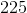Explanation:

We need to arrange the 5 balls in 5 positions: _ _ _ _ _.  The first position can be taken by any of the 5 balls. Then there are 4 balls left to fill the second position, and so on.  Therefore the number of arrangements is 5! = 5 * 4 * 3 * 2 * 1 = 120.

### Example Question #6 : Permutation / Combination

Daisy wants to arrange four vases in a row outside of her garden. She has eight vases to choose from. How many vase arrangements can she make?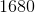Explanation:

For this problem, since the order of the vases matters (red blue yellow is different than blue red yellow), we're dealing with permutations.

Withselections made from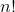potential options, the total number of possible permutations(order matters) is: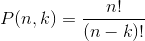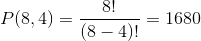### Example Question #2 : Permutation / Combination

What is the minimum amount of handshakes that can occur among fifteen people in a meeting, if each person only shakes each other person's hand once?

105

250

210

32,760

105

Explanation:

This is a combination problem of the form “15 choose 2” because the sets of handshakes do not matter in order.  (That is, “A shakes B’s hand” is the same as “B shakes A’s hand.”)  Using the standard formula we get: 15!/((15 – 2)! * 2!) = 15!/(13! * 2!) = (15 * 14)/2 = 15 * 7 = 105.

### Example Question #3 : Permutation / Combination

There are 20 people eligible for town council, which has three elected members.

Quantity A

The number of possible combinations of council members, presuming no differentiation among office-holders.

Quantity B

The number of possible combinations of council members, given that the council has a president, vice president, and treasurer.

The quantities are equal.

Quantity B is greater.

The relationship cannot be determined from the information given.

Quantity A is greater.

Quantity B is greater.

Explanation:

This is a matter of permutations and combinations. You could solve this using the appropriate formulas, but it is always the case that you can make more permutations than combinations for all groups of size greater than one because the order of selection matters; therefore, without doing the math, you know that B must be the answer.

### Example Question #3 : Permutation / Combination

Joe has a set of 10 books that he hasn't yet read. If he takes 3 of them on vacation, how many possible sets of books can he take?

120

240

None of these

720

1145

120

Explanation:

He can choose from 10, then 9, then 8 books, but because order does not matter we need to divide by 3 factorial

(10 * 9 * 8) ÷ (3 * 2 * 1) = 720/6 = 120

### Example Question #10 : Permutation / Combination

How many different license passwords can one make if said password must contain exactly 6 characters, two of which are distinct numbers, another of which must be an upper-case letter, and the remaining 3 can be any digit or letter (upper- or lower-case) such that there are no repetitions of any characters in the password?

219

231

456426360

619652800

365580800

456426360

Explanation:

Begin by considering the three "hard and fast conditions" - the digits and the one upper-case letter.  For the first number, you will have 10 choices and for the second 9 (since you cannot repeat).  For the captial letter, you have 26 choices.  Thus far, your password has 10 * 9 * 26 possible combinations.

Now, given your remaining options, you have 8 digits, 25 upper-case letters, and 26 lower-case letters (i.e. 59 possible choices).  Since you cannot repeat, you will thus have for your remaining choices 59, 58, and 57 possibilities.

Putting all of this together, you have: 10 * 9 * 26 *59 * 58 * 57 or 456426360 choices.

← Previous 1 3 4 5

Tired of practice problems?

Try live online GRE prep today.